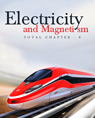Click to Chat

1800-1023-196

+91-120-4616500

CART 0

• 0

MY CART (5)

Use Coupon: CART20 and get 20% off on all online Study Material

ITEM
DETAILS
MRP
DISCOUNT
FINAL PRICE
Total Price: Rs.

There are no items in this cart.
Continue Shopping
`        A capacitor having a capacitance of 100 μF is charged to a potential difference of 24 V. The charging battery is disconnected and the capacitor is connected to another battery of emf 12V with the positive plate of the capacitor joined with the positive terminal of the battery. (a) Find the charges on the capacitor before and after the reconnection. (b) Find the charge flown through the 12 V battery. (c) Is work done by the battery or is it done on the battery? Find its magnitude. (d) Find the decrease in electrostatic field energy. (e) Find the heat developed during the flow of charge after reconnection. `
5 years ago

```							Sol. (a) Before reconnection
C = 100 μf V = 24 V
q = CV = 2400 μc (Before reconnection)
After connection
When C = 100 μf V = 12 V
q = CV = 1200 μc (After connection)
(b) C = 100, V = 12 V
∴ q = CV = 1200 v

(c) We know V = W/q
W = vq = 12 × 1200 = 14400 J = 14.4 mJ
The work done on the battery.
(d) Initial electrostatic field energy Ui = (1/2) CV base 1^2
Final Electrostatic field energy Uf = (1/2) CV base 2^2
∴Decrease in Electrostatic
Field energy = (1/2) CV base 1^2 – (1/2) CV base 2^2
= (1/2) C(V base 1^2 – V base 2^2) = (1/2) × 100(576 –144) = 21600J
∴ Energy = 21600 j = 21.6 mJ
(e)After reconnection
C = 100 μc, V = 12 v
∴ The energy appeared = (1/2) CV^2 = (1/2) × 100 × 144 = 7200 J = 7.2 mJ
This amount of energy is developed as heat when the charge flow through the capacitor.

```
5 years ago
Think You Can Provide A Better Answer ?

## Other Related Questions on Electromagnetic Induction

View all Questions »### Course Features

• 731 Video Lectures
• Revision Notes
• Previous Year Papers
• Mind Map
• Study Planner
• NCERT Solutions
• Discussion Forum
• Test paper with Video Solution### Course Features

• 57 Video Lectures
• Revision Notes
• Test paper with Video Solution
• Mind Map
• Study Planner
• NCERT Solutions
• Discussion Forum
• Previous Year Exam Questions